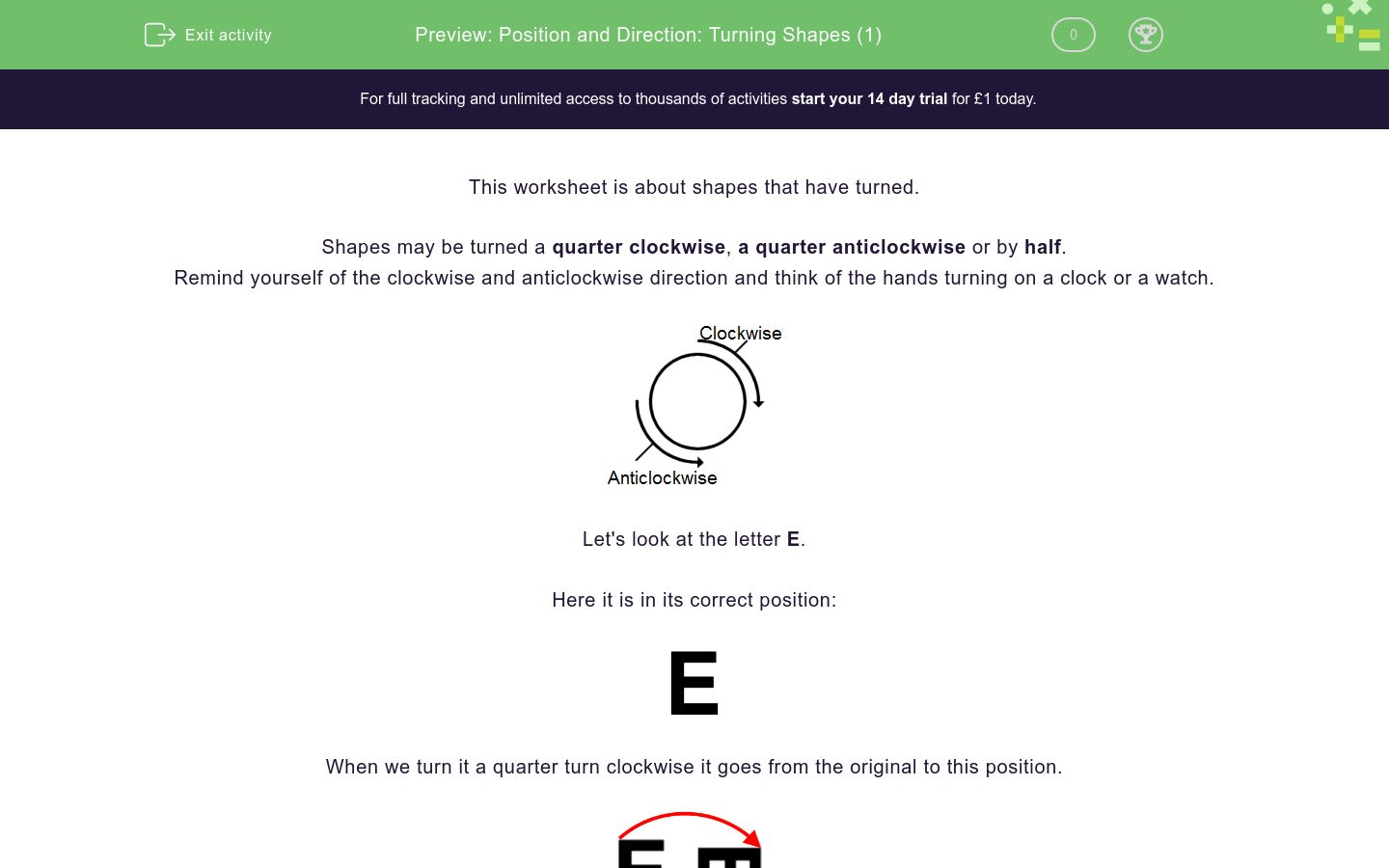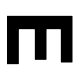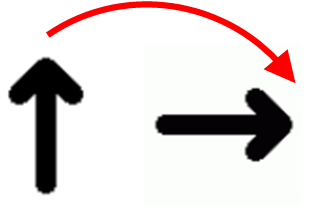# Position and Direction: Turning Shapes (1)

In this worksheet, students say how far round a shape has turned using words such as quarter, half, clockwise and anticlockwise.Key stage:  KS 1

Curriculum topic:   Geometry: Position and Direction

Curriculum subtopic:   Use Language of Position/Direction

Difficulty level:### QUESTION 1 of 10

This worksheet is about shapes that have turned.

Shapes may be turned a quarter clockwise, a quarter anticlockwise or by half.

Remind yourself of the clockwise and anticlockwise direction and think of the hands turning on a clock or a watch.Let's look at the letter E.

Here it is in its correct position:When we turn it a quarter turn clockwise it goes from the original to this position.When we turn it a half turn it goes from the original to this position.

For half turns, we can go clockwise or anticlockwise – it doesn't matter!When we turn it a quarter turn anticlockwise it goes from the original to this position.Describe how the letter E has turned.quarter clockwise

quarter anticlockwise

half

Describe how the letter E has turned.quarter clockwise

quarter anticlockwise

half

Describe how the shapes have turned.quarter clockwise

quarter anticlockwise

half

Describe how the shapes have turned.quarter clockwise

quarter anticlockwise

half

Describe how the shapes have turned.quarter clockwise

quarter anticlockwise

half

Describe how the shapes have turned.quarter clockwise

quarter anticlockwise

half

Describe how the shapes have turned.quarter clockwise

quarter anticlockwise

half

Describe how the shapes have turned.quarter clockwise

quarter anticlockwise

half

Describe how the shapes have turned.quarter clockwise

quarter anticlockwise

half

Describe how the shapes have turned.quarter clockwise

quarter anticlockwise

half

• Question 1

Describe how the letter E has turned.quarter clockwise
EDDIE SAYS• Question 2

Describe how the letter E has turned.half
EDDIE SAYS
This is half a turn clockwise or anticlockwise.
• Question 3

Describe how the shapes have turned.quarter clockwise
EDDIE SAYS• Question 4

Describe how the shapes have turned.half
EDDIE SAYS
This is half a turn clockwise or anticlockwise.
• Question 5

Describe how the shapes have turned.quarter anticlockwise
EDDIE SAYS
The shape has turned anticlockwise.
• Question 6

Describe how the shapes have turned.quarter anticlockwise
EDDIE SAYS
The arrow has turned anticlockwise.
• Question 7

Describe how the shapes have turned.half
EDDIE SAYS
This is half a turn clockwise or anticlockwise.
• Question 8

Describe how the shapes have turned.quarter clockwise
EDDIE SAYS• Question 9

Describe how the shapes have turned.half
EDDIE SAYS
This is half a turn clockwise or anticlockwise.
• Question 10

Describe how the shapes have turned.half
EDDIE SAYS
This is half a turn clockwise or anticlockwise.
---- OR ----

Sign up for a £1 trial so you can track and measure your child's progress on this activity.

### What is EdPlace?

We're your National Curriculum aligned online education content provider helping each child succeed in English, maths and science from year 1 to GCSE. With an EdPlace account you’ll be able to track and measure progress, helping each child achieve their best. We build confidence and attainment by personalising each child’s learning at a level that suits them.

Get started